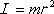The moment of inertia (a.k.a. rotational inertia) of a point mass, with respect to rotation about an axis that is a distance r from the point mass, is the mass m of the point mass times the square of the distance of the point mass from the axis of rotation.

Note 1: It may be difficult to conceive of a point mass in rotation about an axis of rotation some distance from the point mass. It would seem that the point mass is going around in a circle rather than spinning. It helps to imagine a massless rod extending from the axis of rotation to the point mass with the point mass fixed to the end of the rod. Then one can think of the moment of inertia as the moment of inertia of the massless rod with the point mass fixed to the end of the rod. Such an object would be in rotational motion, spinning around on the axis of rotation like the second hand spins around on a clock. The moment of inertia of such an object with respect to its axis of rotation would be I = mr2 and the moment of inertia of such an object is what we mean by the moment of inertia of the point mass (because the massless rod makes no contribution to the moment of inertia).

Note 2: For the moment of inertia to be exactly mr2, the point mass really has to be a point mass, having mass, but no extent in space. For an object, such as a small metal ball, whose diameter is small compared to the distance from the axis of rotation to the center of the object, I = mr2 is a good approximation. (It is important to realize that the r in such a case would be the large distance from the axis of rotation to the center of the ball, not the small radius of the ball.)